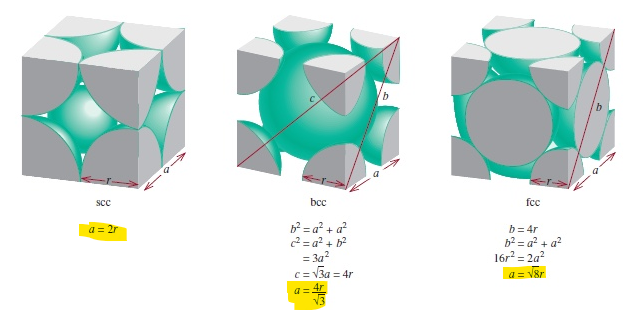# Problem: At room temperature and pressure RbI crystallizes with the NaCl-type structure.Use ionic radii to predict the length of the cubic unit cell edge.

###### FREE Expert Solution

We’re being asked to determine the length of the cubic unit cell edge of RbI using its ionic radii.

In calculating for the unit cell edge length, we may refer to the formula given in the figure below:where a is the cell edge, b is the diagonal length and r is the radius.

Since RbI forms a NaCl-type of structure at room pressure and temperature, it is a face-centered cubic.

We will use the formulas for fcc and do the following steps to solve the problem:

Step 1: Calculate the diagonal

Step 3: Calculate the cell length

85% (267 ratings)###### Problem Details

At room temperature and pressure RbI crystallizes with the NaCl-type structure.

Use ionic radii to predict the length of the cubic unit cell edge.